# MP Board Class 8th Maths Solutions Chapter 1 Rational Numbers Ex 1.1

In this article, we will share MP Board Class 8th Maths Solutions Chapter 1 Rational Numbers Ex 1.1 Pdf, These solutions are solved subject experts from the latest edition books.

## MP Board Class 8th Maths Solutions Chapter 1 Rational Numbers Ex 1.1

Mp Board Class 8 Maths Chapter 1 Question 1.
Using appropriate properties, find.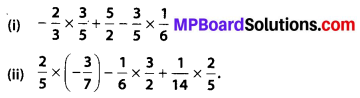Solution: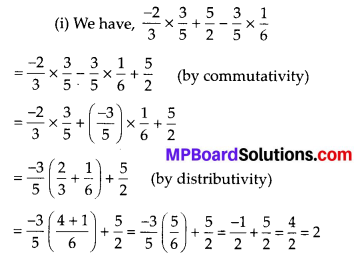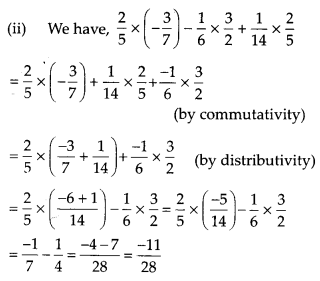Mp Board Class 8 Maths Solutions English Medium Question 2.
Write the additive inverse of each of the following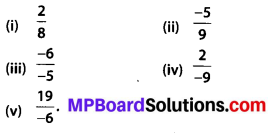Solution: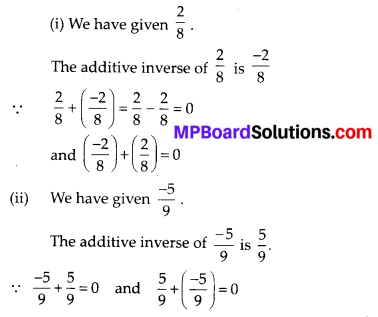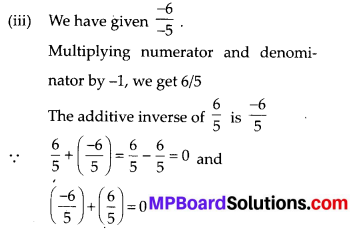(iv) We have given $$\frac{2}{-9}$$
Multiplying numerator and denominator by -1, we get $$\frac{2}{-9}$$
The additive inverse of $$\frac{2}{-9}$$ is $$\frac{2}{9}$$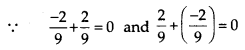(v) We have $$\frac{19}{-6}$$
Multiplying numerator and denominator by -1, we get $$\frac{-19}{6}$$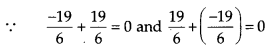Class 8 Maths Chapter 1 Mp Board Question 3.
Verify that -(-x) = x for
(i) x = $$\frac{11}{15}$$
(ii) x = $$-\frac{13}{17}$$
Solution: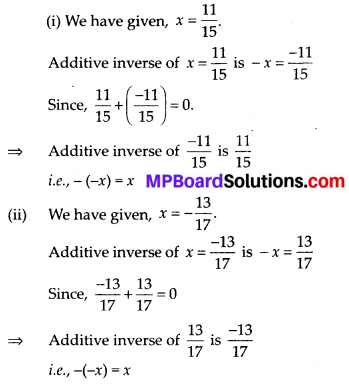Mp Board Solution Class 8 Maths Chapter 1 Question 4.
Find the multiplicative inverse of the following.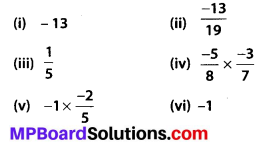Solution:
(i) We have given, -13
The multiplicative inverse of -13 is $$\left(\frac{-1}{13}\right)$$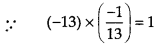(ii) We have given, $$\frac{-13}{19}$$
The multiplicative inverse of is $$\frac{-13}{19}$$ is $$\frac{-19}{13}$$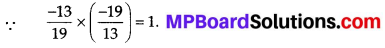(iii) We have given, $$\frac{1}{5}$$
The multiplicative inverse of $$\frac{1}{5}$$ is 5.
∵ $$\frac{1}{5} \times 5=1$$

(iv) We have given, $$\frac{-5}{8} \times \frac{-3}{7}$$
The multiplicative inverse of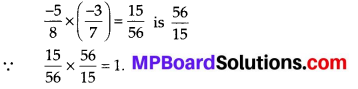(v) We have given, $$-1 \times \frac{-2}{5}$$
The multiplicative inverse of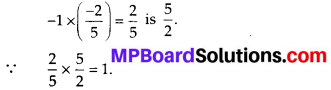(vi) We have given, -1.
The multiplicative inverse of -1 is -1.
∵ (-1) × (-1) = 1.

Mp Board Class 8th Maths Chapter 1 Question 5.
Name the property under multiplication used in each of the following.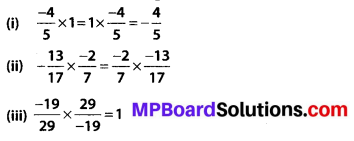Solution:
(i) We have given, $$\frac{-4}{5} \times 1=1 \times \frac{-4}{5}=\frac{-4}{5}$$
i.e., 1 is the multiplicative identity. Thus, it is a identity property under multiplication
(ii) We have given, $$\frac{-13}{17} \times \frac{-2}{7}=\frac{-2}{7} \times \frac{-13}{17}$$, which shows the commutativity.
Thus, it is a commutative property under multiplication.
(iii) We have given $$\frac{-19}{29} \times \frac{29}{-19}=1$$, which shows that $$\frac{29}{-19}$$ is a multiplicative inverse of $$\left(\frac{-19}{29}\right)$$
Thus, it is a inverse property under multiplication.

Mp Board Class 8th Maths Solutions English Medium Question 6.
Multiply $$\frac{6}{13}$$ by the reciprocal of $$\frac{-7}{16}$$.
Solution: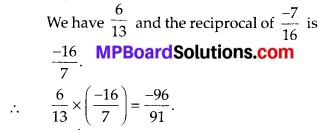Mp Board 8th Class Maths Book Solutions Question 7.
Tell what property allows you to compute $$\frac{1}{3} \times\left(6 \times \frac{4}{3}\right) \text { as }\left(\frac{1}{3} \times 6\right) \times \frac{4}{3}$$
Solution: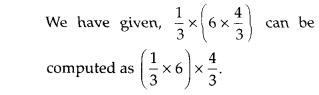Above property is associativity.
[ ∵ a × (b × c) = (a × b) × c]

Maths Class 8 Mp Board Question 8.
Is $$\frac{8}{9}$$ the multiplicative inverse of $$-1 \frac{1}{8}$$ ? Why or why not?
Solution:
We have given a fraction $$\frac{8}{9}$$ and $$-1 \frac{1}{8}=\frac{-9}{8}$$
No, $$\frac{-9}{8}$$ is not a multiplicative inverse of $$\frac{8}{9}$$ because $$\frac{8}{9} \times\left(\frac{-9}{8}\right)=-1 \neq 1$$

Mp Board Class 8 Maths Solutions Question 9.
Is 0.3 the multiplicative inverse of $$3 \frac{1}{3}$$ ? Why or why not?
Solution: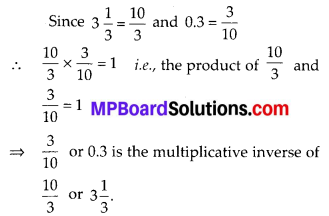Mp Board Class 8 Maths Solution English Medium Question 10.
Write.
(i) The rational number that does not have a reciprocal.
(ii) The rational numbers that are equal to their reciprocals.
(iii) The rational number that is equal to its negative.
Solution:
(i) 0 is the rational number, which does not have a reciprocal.
(ii) 1 and (-1) are the rational numbers, that are equal to their reciprocals.
(iii) 0 is the rational number that is equal to its negative.

Mp Board Solution Class 8 Maths Question 11.
Fill in the blanks.
(i) Zero has …… reciprocal.
(ii) The numbers …… and ……. are their own reciprocals.
(iii) The reciprocals of -5 is ……
(iv) Reciprocal of $$\frac{1}{x}$$, where x ≠ 0 is ……
(v) The product of two rational numbers is always a ……
(vi) The reciprocal of positive rational number is …….
Solution:
(i) Zero has no reciprocal.
(ii) The numbers 1 and -1 are their own reciprocals.
(iii) The reciprocal of -5 is $$\frac{-1}{5}$$ .
(iv) Reciprocal of $$\frac{1}{x}$$, where x ≠ 0 is x.
(v) The product of two rational numbers is always a rational number.
(vi) The reciprocal of positive rational number is positive.